# 1745 How Thorny Is This Rose?

### Today’s Puzzle:

How thorny is this Valentine’s rose? That depends on if you use logic to solve it! It is a Level 5 puzzle, so some of the clues are meant to be tricky. Nevertheless, you can solve it! Just place all the numbers 1 to 10 in both the first column and the top row so that the given clues are the products of the numbers you write. There is only one solution.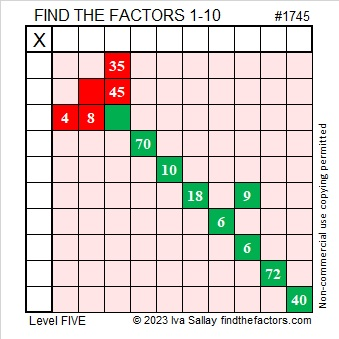Here’s the same puzzle minus the color: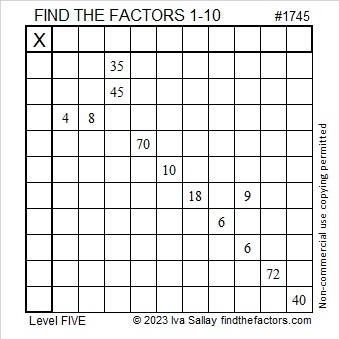### Factors of 1745:

• 1745 is a composite number.
• Prime factorization: 1745 = 5 × 349.
• 1745 has no exponents greater than 1 in its prime factorization, so √1745 cannot be simplified.
• The exponents in the prime factorization are 1 and 1. Adding one to each exponent and multiplying we get (1 + 1)(1 + 1) = 2 × 2 = 4. Therefore 1745 has exactly 4 factors.
• The factors of 1745 are outlined with their factor pair partners in the graphic below.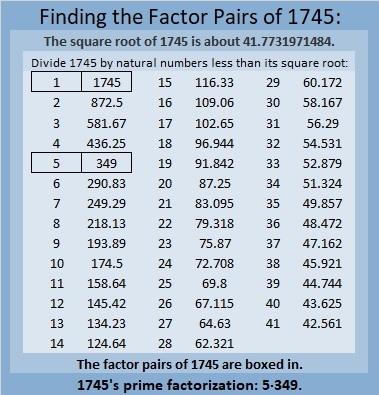### More About the Number 1745:

1745 is the difference of two squares in two different ways:
873² – 872² = 1745, and
177² – 172² = 1745.

1745 is also the sum of two squares in two different ways:
41² + 8² = 1745, and
31² + 28² = 1745.

1745 is the hypotenuse of FOUR Pythagorean triples:
177-1736-1745, calculated from 31² – 28², 2(31)(28), 31² + 28²,
656-1617-1745, calculated from 2(41)(8), 41² – 8², 41² + 8²,
900-1495-1745, which is 5 times (180-299-349)
1047-1396-1745, which is (3-4-5) times 349.

# 1744 XOXO Kisses or Multiplication? XOXO

### Today’s Puzzle:

When I was a little girl, my mother told me that OXOXOX stands for Hugs and Kisses. That would mean that O means “hug” and X means “kiss”, but I think X also means multiplication. It’s almost Valentine’s Day, so in today’s puzzle, it can mean either one.

This is a Level-4 puzzle so the clues don’t come in any particular order. Use logic to place all the numbers 1 to 10 in the first column and in the top row.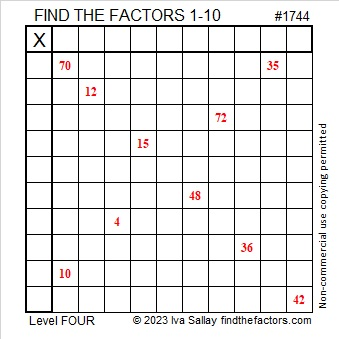### Factors of 1744:

• 1744 is a composite number.
• Prime factorization: 1744 = 2 × 2 × 2 × 2 × 109, which can be written 1744 = 2⁴ × 109.
• 1744 has at least one exponent greater than 1 in its prime factorization so √1744 can be simplified. Taking the factor pair from the factor pair table below with the largest square number factor, we get √1744 = (√16)(√109) = 4√109.
• The exponents in the prime factorization are 4 and 1. Adding one to each exponent and multiplying we get (4 + 1)(1 + 1) = 5 × 2 = 10. Therefore 1744 has exactly 10 factors.
• The factors of 1744 are outlined with their factor pair partners in the graphic below.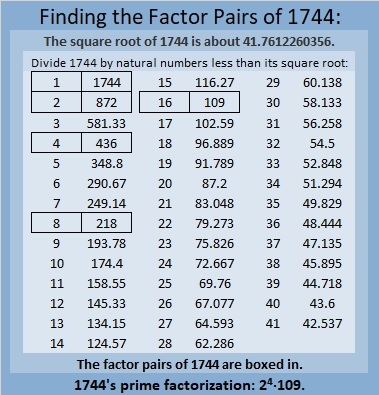### More About the Number 1744:

1744 is the difference of two squares in three ways:
437² – 435² =1744,
220² – 216² =1744, and
113² – 105² =1744.

1744 is also the sum of two squares:
40² + 12² = 1744.

1744 is the hypotenuse of a Pythagorean triple:
960-1456-1744, calculated from 2(40)(12), 40² – 12², 40² + 12².
It is also 16 times (60-91-109).

# 1743 Finding Ways to Transform My Heart

### Today’s Puzzle:

An equation of a unit circle centered at the origin is x² + y² = 1.

If we change just the “y” part of that equation, we can get a lovely heart just in time for Valentine’s Day. Try it yourself by typing the equations into Desmos.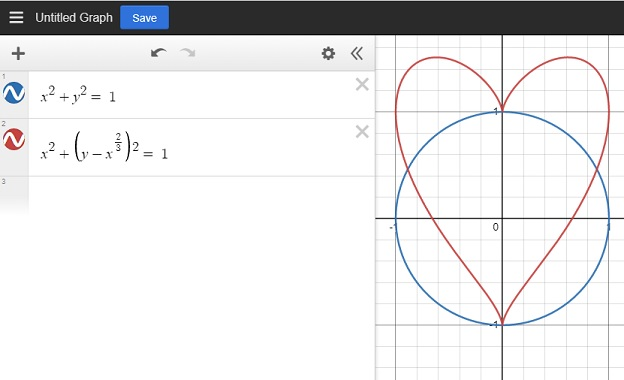There are other mathematical equations for a heart, but this is the one I’m exploring in this post.

I was puzzled over how I could transform that heart. Can I make it bigger, or dilate it? Can I slide it away from the origin or translate it? Can I rotate it? Can I reflect it across the x or y-axis?

These are questions I’d like you to explore as well.

### Heart Dilation:

In this first graphic, I was able to make my heart bigger. What kind of math let me do that? Also, how did I color the inside of some of the hearts? Look at the equations next to the heart and try to figure it out. The concentric hearts are evenly spaced. Do you recognize a pattern in the numbers that made that happen?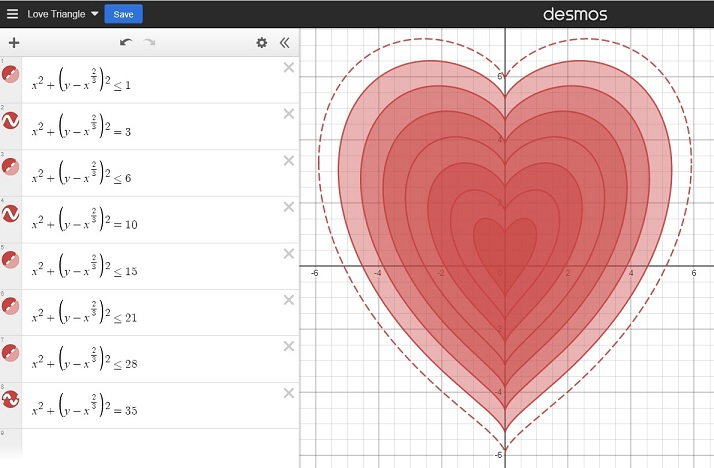### Heart Slide (Translation):

If we changed the center of a circle to (a, -b) instead of the origin, we would slide the whole circle. Here’s how we change the equation of the circle to give it a new center:
(x-a)²+(y+b)² = 1.

Similarly, in the next graphic, I was able to slide my heart away from the origin. How did I do that? Look at the equations to see how.### Heart Rotation:

A circle looks the same no matter how it is rotated, but the same isn’t true for a heart. Look at the equations below. How was I able to rotate my heart around the origin?### Heart Reflection:

Since a heart is symmetric, its reflection across the x-axis doesn’t look that interesting to me. Instead, I created a double heart that I reflected across both the x-axis and the y-axis: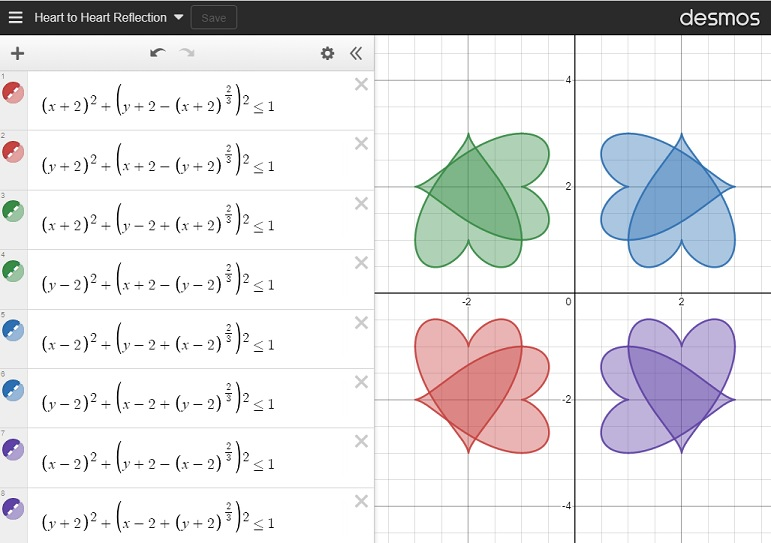### Just for Fun:

Next, I was curious about what would happen if I changed the exponents on the outside of the parenthesis, so I changed a 2 from my original equation to an 8 in a couple of different places as I moved the heart from left to right. How did changing the exponent affect my heart? I found that as long as the exponent stays even, it still looks a little like a heart.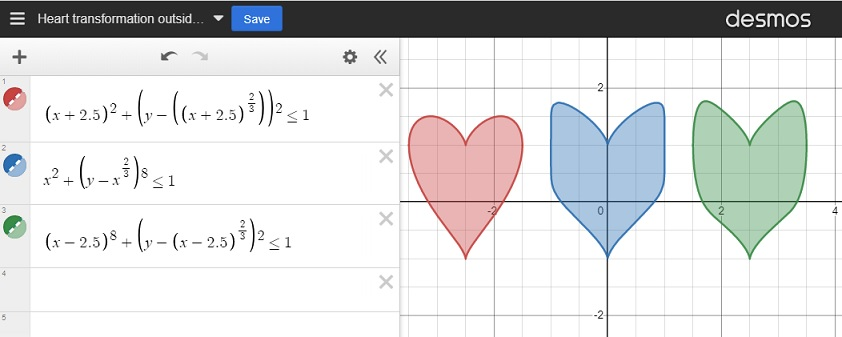I was also curious about what would happen to my heart if I changed the “2/3” to a different fraction. I used fractions less than one as well as fractions greater than one. For many of my fractions, I used the post number, 1743, as the denominator. As long as the numerator was even and the denominator was odd, the graph still looked mostly like a heart. However, the closer the fraction was to zero, the more it looked like a circle.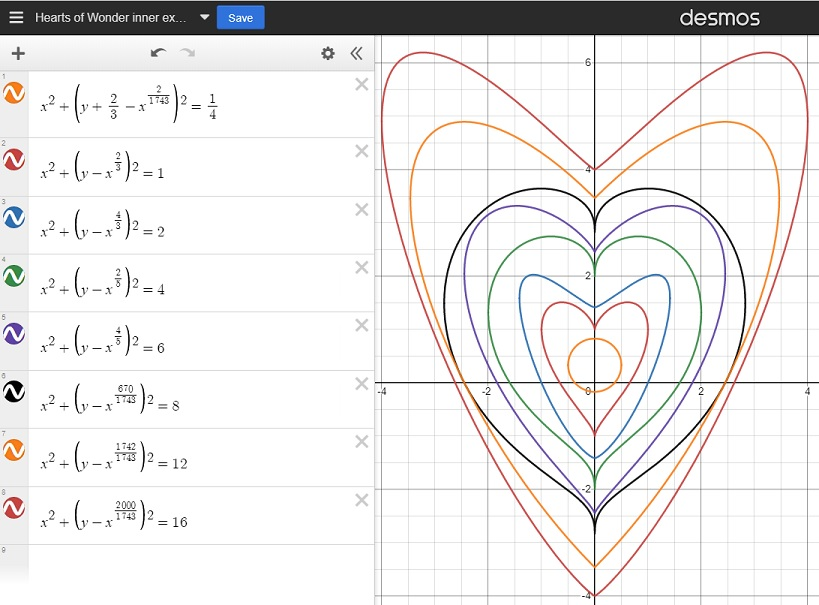Finally, I created this lovely flower using some of what I learned by making these transformations:And for just a little bit more fun, I created a simple but chaotic-looking animation that I’ve titled Hearts in Motion. Enjoy!

I had so much fun exploring this heart in Desmos. Thank you for allowing me to share my excitement with you.

### Factors of 1743:

• 1743 is a composite number.
• Prime factorization: 1743 = 3 × 7 × 83.
• 1743 has no exponents greater than 1 in its prime factorization, so √1743 cannot be simplified.
• The exponents in the prime factorization are 1, 1, and 1. Adding one to each exponent and multiplying we get (1 + 1)(1 + 1)(1 + 1) = 2 × 2 × 2 = 8. Therefore 1743 has exactly 8 factors.
• The factors of 1743 are outlined with their factor pair partners in the graphic below.### More About the Number 1743:

1743 is the difference of two squares in four different ways:
872² – 871² = 1743,
292² – 289² = 1743,
128² – 121² = 1743, and
52² – 31² = 1743.

# 1742 Just How Sharp Do You Think Cupid’s Arrow Is?

### Today’s Puzzle:

This puzzle looks a little like an arrow that Cupid might be aiming your way. I’m not sure how sharp it is, but here’s hoping that it’s not the least bit painful.

Since this is a level-3 puzzle, start with the clues at the top of the puzzle and work your way down cell by cell until you have found all the factors. Each number from 1 to 10 can only appear one time in the first column and one time in the top row. There is only one solution.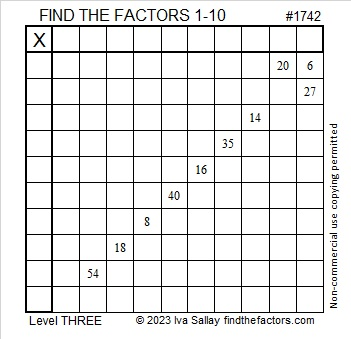### Factors of 1742:

• 1742 is a composite number.
• Prime factorization: 1742 = 2 × 13 × 67.
• 1742 has no exponents greater than 1 in its prime factorization, so √1742 cannot be simplified.
• The exponents in the prime factorization are 1, 1, and 1. Adding one to each exponent and multiplying we get (1 + 1)(1 + 1)(1 + 1) = 2 × 2 × 2 = 8. Therefore 1742 has exactly 8 factors.
• The factors of 1742 are outlined with their factor pair partners in the graphic below.### More About the Number 1742:

1742 is the hypotenuse of a Pythagorean triple:
670-1608-1742 which is (5-12-13) times 134.

1742 looks interesting in some other bases:
It’s 23432 in base 5 because 2(5⁴) + 3(5³) + 4(5²) + 3(5) + 2(1) = 1742,
2345 in base 9 because 2(9³) + 3(9²) + 4(9) + 5(1) = 1742, and
repdigit 222 in base 29 because 2(29² + 29 + 1) = 1742.

# 1741 What Do You Notice About the Remainders?

### Today’s Puzzle:

The table below is a list of the first 280 prime numbers. Below each prime number is its remainder when the prime number is divided by 6. What do you notice about the remainders? What do you wonder?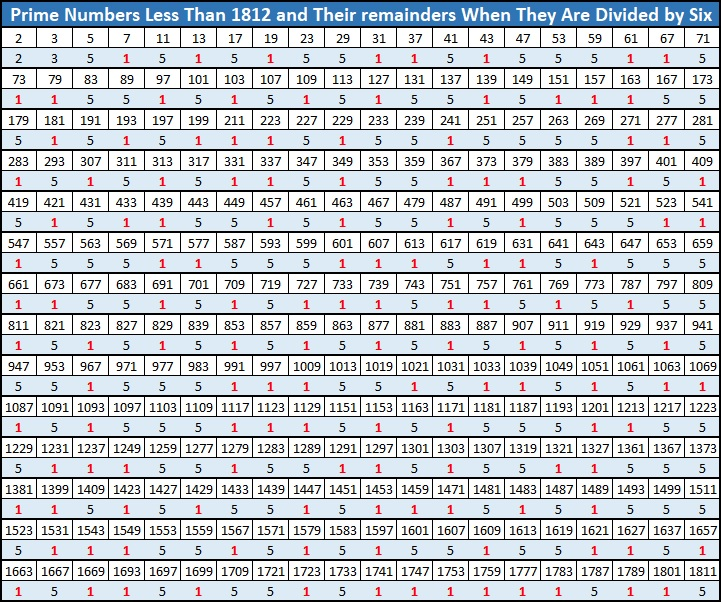Did you notice a fun fact starting with the number 1741? I would like to thank OEIS.org for making me aware of it.

### Factors of 1741:

• 1741 is a prime number.
• Prime factorization: 1741 is prime.
• 1741 has no exponents greater than 1 in its prime factorization, so √1741 cannot be simplified.
• The exponent in the prime factorization is 1. Adding one to that exponent we get (1 + 1) = 2. Therefore 1741 has exactly 2 factors.
• The factors of 1741 are outlined with their factor pair partners in the graphic below.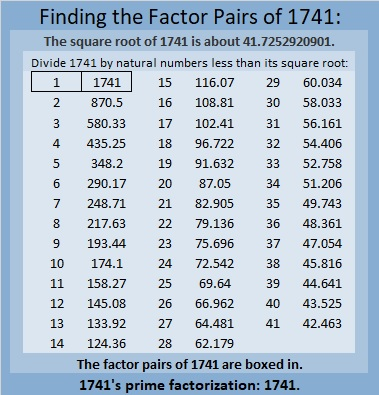How do we know that 1741 is a prime number? If 1741 were not a prime number, then it would be divisible by at least one prime number less than or equal to √1741. Since 1741 cannot be divided evenly by 2, 3, 5, 7, 11, 13, 17, 19, 23, 29, 31, 37, or 41, we know that 1741 is a prime number.

### More About the Number 1741:

1741 is the sum of two squares:
30² + 29² = 1741.

Here’s another way we know that 1741 is a prime number: Since its last two digits divided by 4 leave a remainder of 1, and 30² + 29² = 1741 with 30 and 29 having no common prime factors, 1741 will be prime unless it is divisible by a prime number Pythagorean triple hypotenuse less than or equal to √1741. Since 1741 is not divisible by 5, 13, 17, 29, 37, or 41, we know that 1741 is a prime number.

Since 29 and 30 are consecutive numbers, we have another fun fact: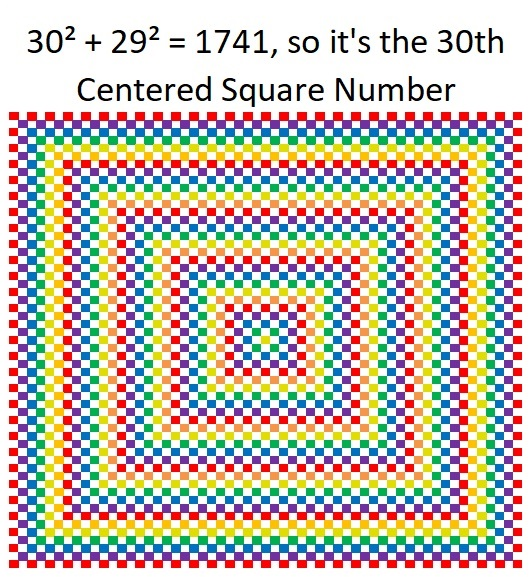And 1741 is the hypotenuse of a Pythagorean triple:
59-1740-1741, calculated from 30² – 29², 2(30)(29), 30² + 29².

1741 is also the difference of two consecutive squares:
871² – 870² = 1741.

# 1740 I Think You’re Going to L♥ve This Multiplication Puzzle

### Today’s Puzzle:

Here’s a heart-shaped level 2 puzzle for you to do. Just place all the numbers from 1 to 10 in both the first column and the top row so that those numbers are the factors of the given clues.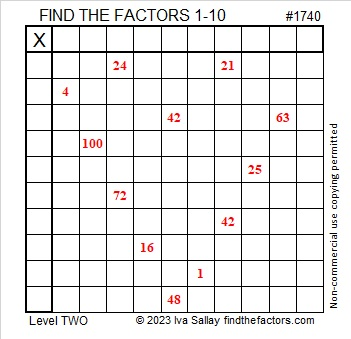Here’s the same puzzle in black and white:### Factors of 1740:

• 1740 is a composite number.
• Prime factorization: 1740 = 2 × 2 × 3 × 5 × 29, which can be written 1740 = 2² × 3 × 5 × 29.
• 1740 has at least one exponent greater than 1 in its prime factorization so √1740 can be simplified. Taking the factor pair from the factor pair table below with the largest square number factor, we get √1740 = (√4)(√435) = 2√435.
• The exponents in the prime factorization are 2, 1, 1, and 1. Adding one to each exponent and multiplying we get (2 + 1)(1 + 1)(1 + 1)(1 + 1) = 3 × 2 × 2 × 2 = 24. Therefore 1740 has exactly 24 factors.
• The factors of 1740 are outlined with their factor pair partners in the graphic below.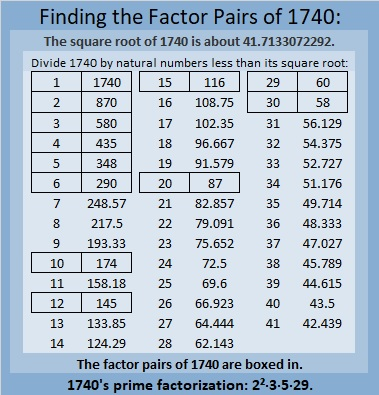### More About the Number 1740:

1740 is the hypotenuse of FOUR Pythagorean triples:
204-1728-1740, which is 3 times (68-576-580),
288-1716-1740, which is 3 times (96-572-580),
1044-1392-1740, which is (3-4-5) times 348, and
1200-1260-1740, which is (20-21-29) times 60.

1740 is palindrome 606 in base 17 because
6(17²) + 0(17) + 6(1) = 1740.

1740 is the difference of two squares in four different ways:
436² – 434² = 1740,
148² – 142² = 1740,
92² – 82² = 1740, and
44² – 14² = 1740.

# 1739 A Gift for a Valentine

### Today’s Puzzle:

Here’s a level 1 Valentine’s gift puzzle to start off the month of February. Place the numbers 1 to 10 in both the first column and the top row so that those numbers are the factors of the given number clues.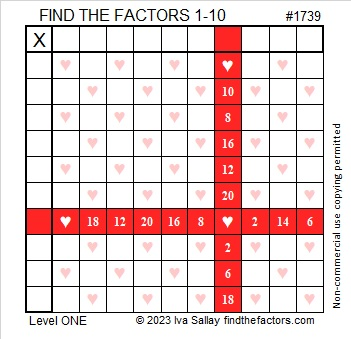And here’s a heartless copy of the same puzzle that may be more printer-friendly.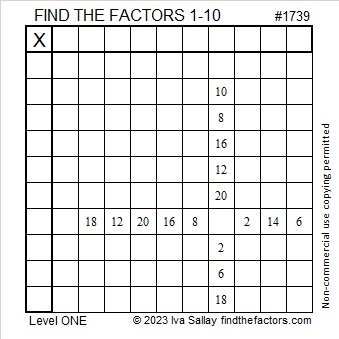### Factors of 1739:

Is 1739 a prime number? It isn’t divisible by 2 or 5.
1 + 7 + 3 + 9 = 20, so it isn’t divisible by 3.
1 – 7 + 3 – 9 = -12, so it isn’t divisible by 11.
√1739 is a little more than 41. Should I try dividing 1739 by every other prime number less than 41? I don’t know divisibility tricks for most of those prime numbers!

Here’s a shortcut I first wrote about in 341 is the smallest composite number that gives a false positive for this Quick Prime Number Test: First I type into my computer’s scientific calculator:
2  xʸ  1739  Mod  1739  =

If the answer on the calculator is anything other than “2”, then it can’t be a prime number. If it is “2”, it very likely is prime. As you can see by the screenshot of my calculator, 1739 is not prime!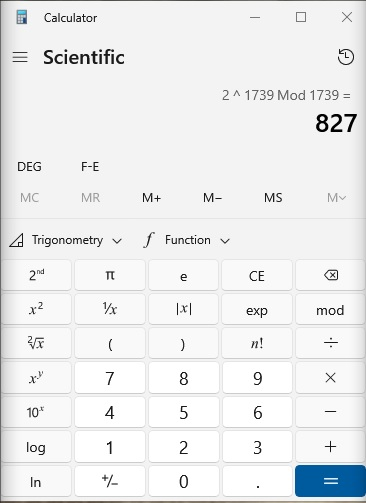• 1739 is a composite number.
• Prime factorization: 1739 = 37 × 47.
• 1739 has no exponents greater than 1 in its prime factorization, so √1739 cannot be simplified.
• The exponents in the prime factorization are 1 and 1. Adding one to each exponent and multiplying we get (1 + 1)(1 + 1) = 2 × 2 = 4. Therefore 1739 has exactly 4 factors.
• The factors of 1739 are outlined with their factor pair partners in the graphic below.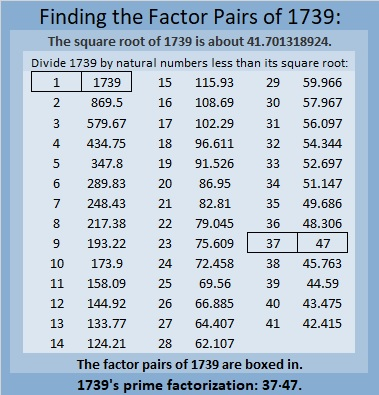### More About the number 1739:

1739 is the difference of two squares in two ways:
870² – 869² = 1739, and
42² – 5² = 1739.

That means 1739 is only 25 numbers away from the next perfect square!

# 1738 Little Surprises Around Every Turn

### Today’s Puzzle:

Is this mystery-level puzzle easy or difficult? I’m not saying. Place all the numbers from 1 to 12 in both the first column and the top row so that those numbers are the factors of the given clues. If you use logic to solve the puzzle, you will probably find some surprises around every turn.### Factors of 1738:

It may surprise you that 1738 is divisible by 11. Why is it? Because
1 – 7 + 3 – 8 = -11, a multiple of 11, which makes 1738 divisible by 11.

• 1738 is a composite number.
• Prime factorization: 1738 = 2 × 11 × 79.
• 1738 has no exponents greater than 1 in its prime factorization, so √1738 cannot be simplified.
• The exponents in the prime factorization are 1, 1, and 1. Adding one to each exponent and multiplying we get (1 + 1)(1 + 1)(1 + 1) = 2 × 2 × 2 = 8. Therefore 1738 has exactly 8 factors.
• The factors of 1738 are outlined with their factor pair partners in the graphic below.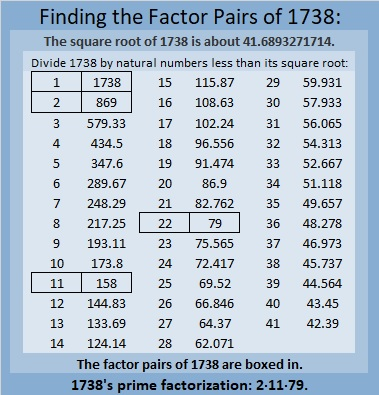### More About the Number 1738:

Maybe you will be surprised by some of these number facts about the number 1738.

As mentioned in the table, 1738 = 2 × 11 × 79. That means 1738 is the short leg in the Pythagorean triple calculated from 2(79)(11), 79² – 11², 79² + 11².

1738 is the sum of eleven consecutive numbers:
153 + 154 + 155 + 156 +157 + 158 + 159 + 160 + 161 + 162 + 163 = 1738.

1738 is the sum of four consecutive numbers:
433 + 434 + 435 + 436 = 1738.

And because four is an even number:
436² – 435² + 434² – 433² = 1738.
Surprised?

1738 is also the sum of the 44 consecutive numbers from 18 to 61.

Consequently,
61² – 60² + 59² – 68² + 57² – 56² + 55² – 54² + 53² – 52² + 51² – 50² + 49² – 48² + 47² – 46² + 45² – 44² + 43² – 42² + 41² – 40² + 39² – 38² + 37² – 36² + 35² – 34² + 33² – 32² + 31² – 30² + 29² – 28² + 27² – 26² + 25² – 24² + 23² – 22² + 21² – 20² + 19² – 18² = 1738. I bet you weren’t expecting that!

But the biggest surprise about 1738 is something I learned from OEIS.org: 1738 is in an equation that uses every digit from 1 to 9 exactly one time:make science GIFs like this at MakeaGif

# 1737 What Logic Will You Use to Solve This Puzzle?

### Today’s Puzzle:

Place each number from 1 to 12 in both the first column and the top row so that those numbers are the factors of the given clues. This is a level 6 puzzle so the logic needed to solve the puzzle will be a little more complicated. Good luck!### Factors of 1737:

1 + 7 + 3 + 7 = 18, a number divisible by nine, so 1718 is divisible by 9.

• 1737 is a composite number.
• Prime factorization: 1737 = 3 × 3 × 193, which can be written 1737 = 3² × 193.
• 1737 has at least one exponent greater than 1 in its prime factorization so √1737 can be simplified. Taking the factor pair from the factor pair table below with the largest square number factor, we get √1737 = (√9)(√193) = 3√193.
• The exponents in the prime factorization are 2 and 1. Adding one to each exponent and multiplying we get (2 + 1)(1 + 1) = 3 × 2 = 6. Therefore 1737 has exactly 6 factors.
• The factors of 1737 are outlined with their factor pair partners in the graphic below.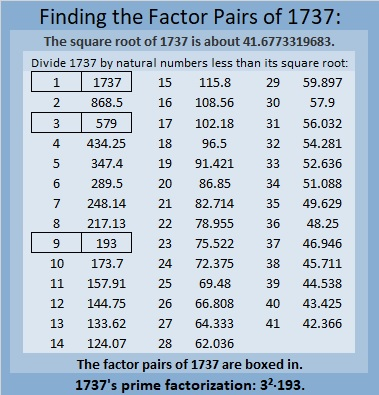### More About the Number 1737:

1737 is the difference of two squares in three ways:
869² – 868² = 1737,
291² – 288² = 1737, and
101² – 92² = 1737.

1737 is the sum of consecutive numbers:
868 + 869 = 1737,
578 + 579 +560 = 1737,
287 + 288 + 289 + 290 + 291 + 292 = 1737,
189 + 190 + 191 + 192 + 193 + 194 + 195 + 196 + 197 = 1737, and
88+89+90+91+92+93+94+95+96+97+98+99+100+101+102+103+104+105=1737.

That first sum uses the same numbers as the first difference of two squares. Check out these square equations!

292² – 291² + 290² – 289² + 288² – 287² = 1737.

105² – 104² + 103² – 102² + 101² – 100² + 99² – 98² + 97² – 96² + 95² – 94² + 93² – 92² + 91² – 90² + 89² – 88² = 1737.

(But since 101² – 92² = 1737, we can conclude that all the rest of the numbers added and subtracted will give us zero!)

1737 is also the sum of two squares:
36² + 21² = 1737.

1737 is the hypotenuse of a Pythagorean triple:
855-1512-1737 calculated from 36² – 21², 2(36)(21), 36² + 21².
That triple is also 9 times (95-168-193).

That Pythagorean triple means that
855² + 1512² = 1737².

From OEIS.org, we learn that when we add up these three consecutive squares,
1736² + 1737² + 1738² we get a palindrome, specifically, 9051509. Cool!

1737² = 1736² + 1734² – 1733² + 1732² – 1731² – 1729² + 1728².

# 1736 Fun With Magic Squares

### Today’s Puzzle is a 7×7 Magic Square:

1736 is the sum of seven consecutive numbers:
245 + 246 + 247 + 248 + 249 + 250 + 251 = 1736.

That means 1736 is the magic sum of a 7×7 Magic Square with the numbers from that sum running along the diagonal. Can you write the rest of the consecutive numbers from 224 to 272 to complete the magic square?If you need some help, trace with your finger while you count from 224 to 272 and see where the numbers fall diagonally on this completed magic square. You should notice that you always place the next number diagonally above to the right unless you can’t, in which case you place the next number directly under your last entry. Sometimes you will have to leave the square and get back on it on the opposite side of the square to maintain the diagonal.

After studying the pattern, try to do it yourself. This excel sheet has a place for you to type the numbers for this magic square and every other magic square discussed in this post. The spreadsheet will even keep a running sum of each column, row, and diagonal as you type in the numbers: 12 Factors 1730-1738.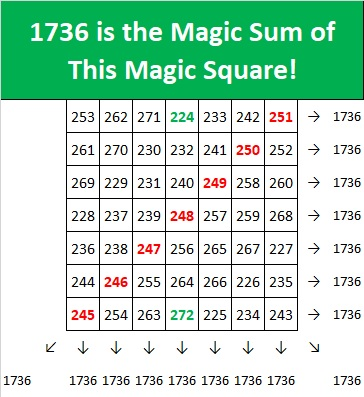The current year is also divisible by seven, and consequently
286 + 287 + 288 + 289 + 290 + 291 + 292 = 2023.
Can you make a 7×7 Magic Square with 2023 as the magic sum?Here is that completed square as well. It follows the same mostly diagonal path as the previous completed magic square:### 17×17 Magic Square:

2023 is divisible by 17, and consequently

111 + 112 + 113 + 114 + 115 + 116 + 117 + 118 + 119 + 120 + 121 + 122 + 123 + 124 + 125 + 126 + 127 = 2023.

All that means that 2023 is also the magic sum of a 17×17 Magic Square, but we also have to use some negative numbers to make it. Trace the numbers beginning with -25 and notice the same diagonal pattern in this magic square.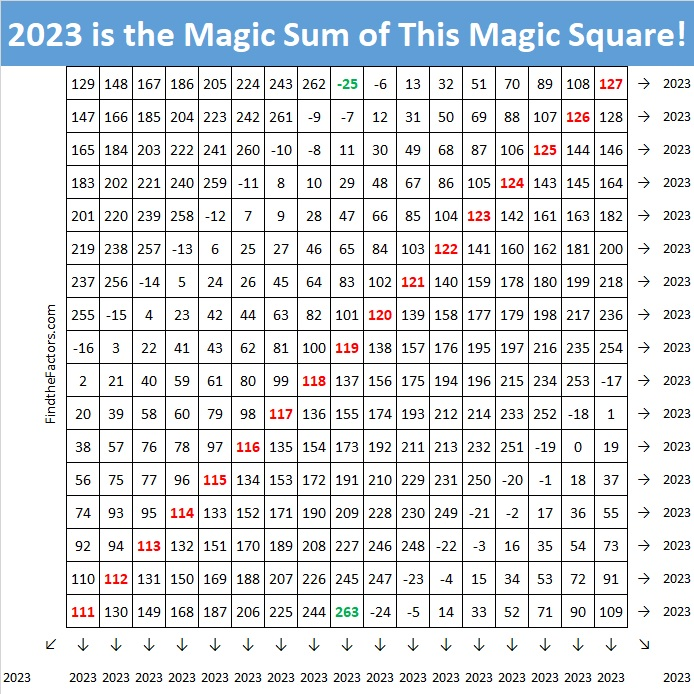### 14×14 Magic Square:

It’s a bit trickier, but since 2023 is divisible by seven but not by 14, it is also the magic sum of a 14×14 Magic Square. To make it start off by dividing the 14×14 square into four 7×7 squares. Place the numbers from 47 to 95 in the top left square, the numbers from 96 to 144 in the bottom right square, the numbers from 145 to 193 in the top right square, and the numbers from 194 to 242 in the bottom left square.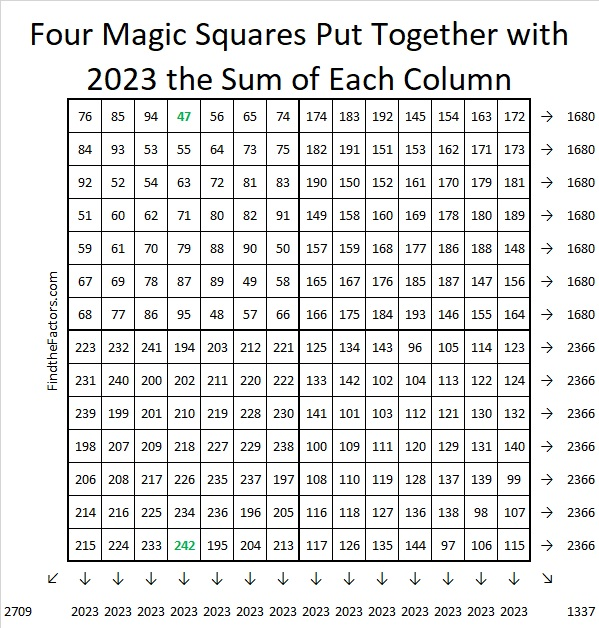Notice that all of the columns show our desired magic sum, but none of the rows or diagonals do. We need to switch some of the numbers to fix that. Switch the shaded areas indicated below with their corresponding darker shaded areas: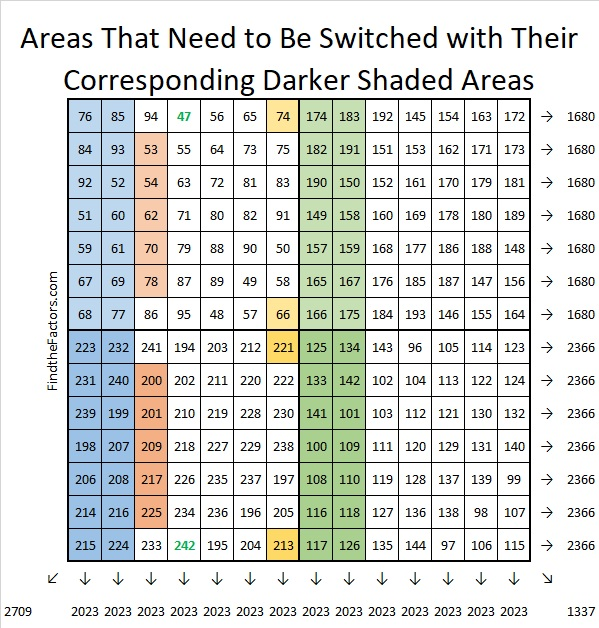And you will successfully create this beautiful magic square where every row, column, and diagonal equals 2023.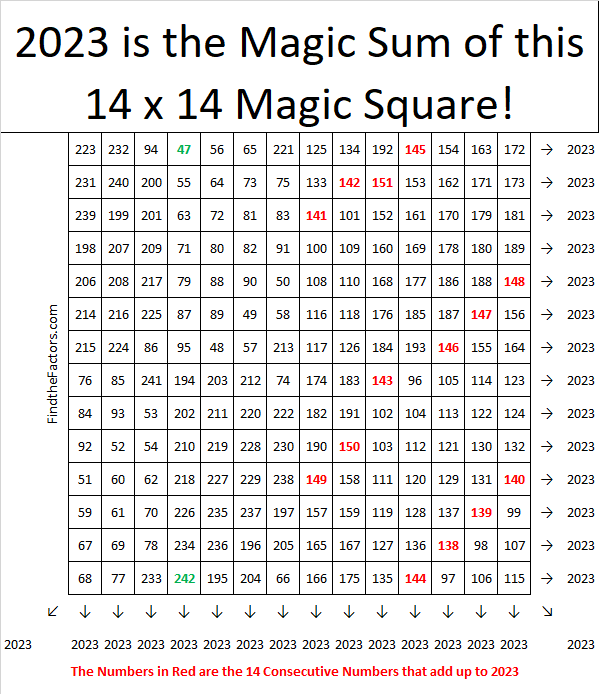Notice that the 14 consecutive numbers that add up to 2023 are all over the square.
138+139+140+141+142+143+144+145+146+147+148+149+150+151 = 2023

Any other magic square for 2023 would be too big and have so many negative numbers.

### 16×16 Magic Square:

1736 is divisible by 8, but not by 16, so there are 16 consecutive numbers that add up to 1736:

101 + 102 + 103 + 104 + 105 + 106 + 107 + 108 + 109 + 110 + 111 + 112 + 113 + 114 + 115 + 116 + 117 = 1736.

Making a 16×16 Magic Square will be a bit tedious, but so satisfying. Start by placing the numbers from -19 to 236 in order from left to right filling in each 4×4 square before moving onto the next 4×4 square, as illustrated below: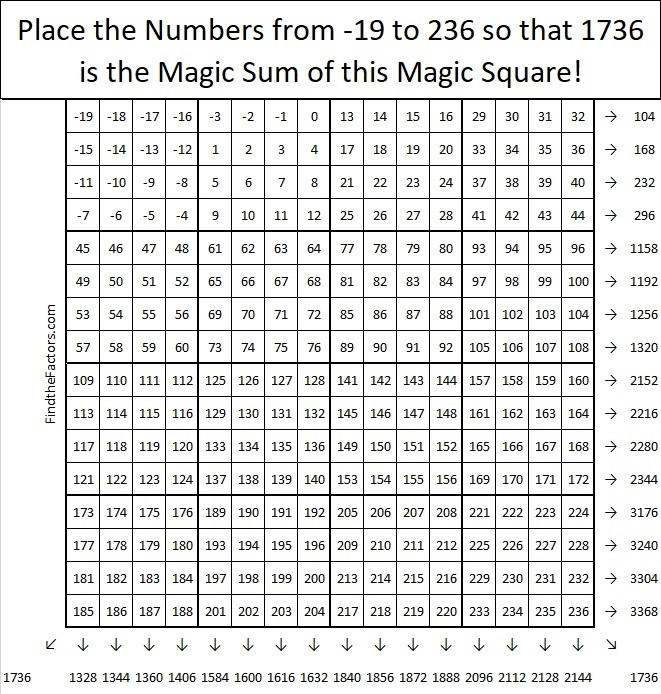Notice that only the diagonals show the desired sum of 1736.

Next, we want to flip the diagonals of each small 4×4 square, as shown below.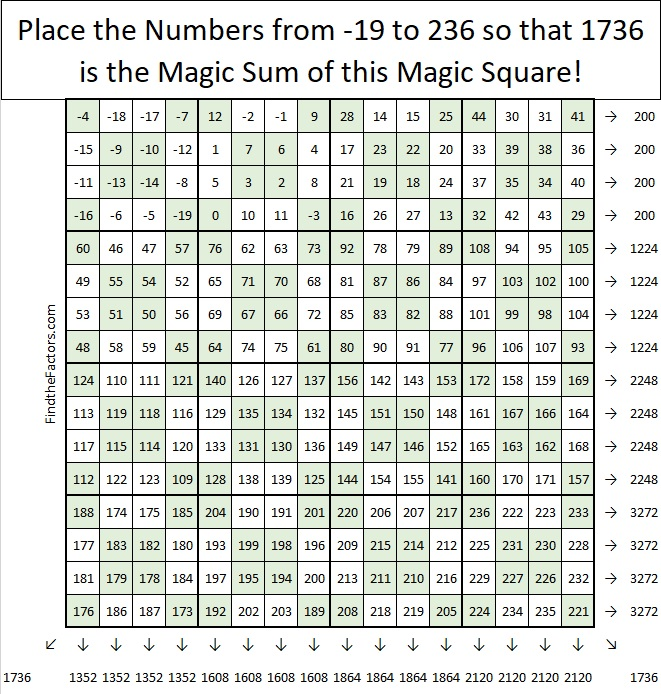The diagonals still have the correct sum, and look at those sets of four columns or four rows that have equal sums! Now think of the whole square as one big 4×4 Magic Square, and flip its diagonals as shown below:Oh, it is a thing of beauty, don’t you agree?

### Factors of 1736:

• 1736 is a composite number.
• Prime factorization: 1736 = 2 × 2 × 2 × 7 × 31, which can be written 1736 = 2³ × 7 × 31.
• 1736 has at least one exponent greater than 1 in its prime factorization so √1736 can be simplified. Taking the factor pair from the factor pair table below with the largest square number factor, we get √1736 = (√4)(√434) = 2√434.
• The exponents in the prime factorization are 3,1 and 1. Adding one to each exponent and multiplying we get (3 + 1)(1 + 1) (1 + 1) = 4 × 2 × 2 = 16. Therefore 1736 has exactly 16 factors.
• The factors of 1736 are outlined with their factor pair partners in the graphic below.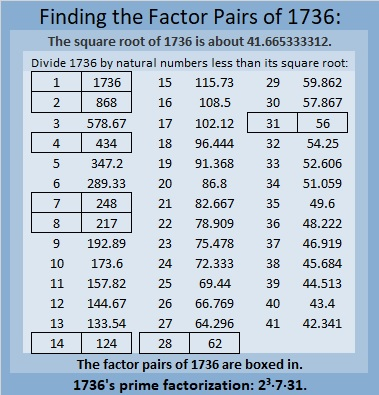### More About the Number 1736:

1736 is the difference of two squares in four different ways:
435² – 433² = 1736,
219² – 215² = 1736,
69² – 55² = 1736, and
45² – 17² = 1736.